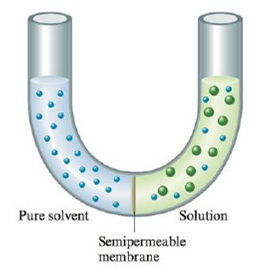Chapter 11, Problem 104AE

Chapter
Section
Textbook Problem

# Consider the following:What would happen to the level of liquid in the two arms if the semipermeable membrane separating the two liquids were permeable toa. H2O (the solvent) only?b. H2O and solute?

(a)

Interpretation Introduction

Interpretation: The Osmosis and Osmotic pressure of given solute and solvent has to be explained.

Concept introduction: A solution and clean solvent are split by a semi-permeable membrane,

This allows solvent except solute molecules to pass from side to side. While time passes,

the quantity of the solution increases and so as to of the solvent decreases. This run of

solvent into the solution from side to side the semi-permeable membrane is called osmosis.

Ultimately the liquid levels stop altering, representative that the system has reached

Equilibrium. For the reason that the liquid levels are different at this point, there is a superior hydrostatic pressure on the solution than on the clean solvent. This overload pressure is

called the osmotic pressure.

The osmotic pressure on solution concentration is represented by the equation

π= MRTwhereπis the osmotic pressure in atmospheres,Mis the molarity of the solution,Risthe gas law constant, and T is the Kelvin temperature.

Explanation

Water only

Figure-1

The water would travel from left to right (to the side with the solution). At first, the level of water in the left arm would go downhill, and the level in the right arm would go up and about...

(b)

Interpretation Introduction

Interpretation: The Osmosis and Osmotic pressure of given solute and solvent has to be explained.

Concept introduction: A solution and clean solvent are split by a semi-permeable membrane,

This allows solvent except solute molecules to pass from side to side. While time passes,

the quantity of the solution increases and so as to of the solvent decreases. This run of

solvent into the solution from side to side the semi-permeable membrane is called osmosis.

Ultimately the liquid levels stop altering, representative that the system has reached

Equilibrium. For the reason that the liquid levels are different at this point, there is a superior hydrostatic pressure on the solution than on the clean solvent. This overload pressure is

called the osmotic pressure.

The osmotic pressure on solution concentration is represented by the equation

π= MRTwhereπis the osmotic pressure in atmospheres,Mis the molarity of the solution,Risthe gas law constant, and T is the Kelvin temperature.

### Still sussing out bartleby?

Check out a sample textbook solution.

See a sample solution

#### The Solution to Your Study Problems

Bartleby provides explanations to thousands of textbook problems written by our experts, many with advanced degrees!

Get Started